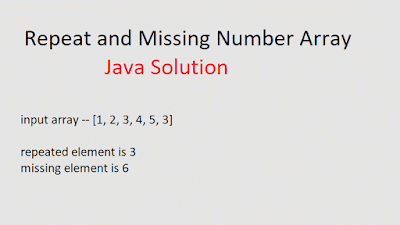## Posts

Showing posts from March, 2019

### Repeat and Missing Number Array - The Coding ShalaHome >> Interview Questions >> Repeat and missing Number Array Repeat and Missing Number Array Solution Problem Statement:: You are given a read-only array of n integers from 1 to n. Each integer appears exactly once except A which appears twice and B which is missing. Return A and B. Note that in your output A should precede B. Example: Input:[3 1 2 5 3] Output:[3, 4] A = 3, B = 4 Solution 1: (Java) public class Solution { public ArrayList < Integer > repeatedNumber ( final List < Integer > A ) { int n = A . size (); ArrayList < Integer > C = new ArrayList < Integer >(); int [] arr = new int [ n + 1 ]; for ( int i = 0 ; i < n + 1 ; i ++) arr [ i ]= 0 ; for ( int i = 0 ; i < A . size (); i ++) arr [ A . get ( i )]++; int A1 = 0 , B = 0 ; for ( int i = 1 ; i < n + 1 ; i ++){ if